### Integers - Solutions

CBSE Class –VII Mathematics

NCERT Solutions
Chapter 1 Integers
(Ex. 1.1)

Question 1. Following number line shows the temperature in degree Celsius {${}^{\circ }$C} at different places on a particular day: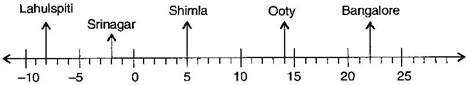(a) Observe this number line and write the temperature of the places marked on it.
(b) What is the temperature difference between the hottest and the coldest places among the above?
(c) What is the temperature difference between Lahulspiti and Srinagar?
(d) Can we say temperature of Srinagar and Shimla taken together is less than the temperature at Shimla? Is it also less than the temperature at Srinagar?

Answer: (a) The temperature of the places marked on it is:

 Places Temperature Bangalore 22o C Ooty 14o C Shimla 5o C Srinagar -2o C Lahulspiti -8o C

(b) The temperature of the hottest place Bangalore = 22C

The temperature of the coldest place Lahulspiti = -8o C

Difference =  22C - ( -8o C) = 22C + 8o C  = 30C

(c)The temperature of Srinagar = -2C

The temperature of Lahulspiti = -8C

Difference = -2C - (-8C) = -2C + 8C = 6C

(d)The temperature of Srinagar and Shimla = -2C + 5C = 3C

The temperature at Shimla = 5C

Therefore, 3C < 5C

Thus, temperature of Srinagar and Shimla taken together is less than the temperature at Shimla.

Now, Temperature of Srinagar = -2C

Therefore, 3oC > -2C

No, it is not less than the temperature at Srinagar.

Question 2. In a quiz, positive marks are given for correct answers and negative marks are given for incorrect answers. If jack’s scores in five successive rounds were 25, – 5, – 10, 15 and 10, what was his total at the end?

Answer: Jack’s scores in five successive rounds are 25, - 5, - 10,15 and 10.

Total marks got by Jack = 25 + (- 5)  + (-10) + 15 + 10 = 25 - 5 - 10 +15 + 10

= 25 – 15 + 25 = 35

Thus, 35 marks are got by Jack in a quiz.

Question 3. At Srinagar temperature was -5oC on Monday and then it dropped by 2oC on Tuesday. What was the temperature of Srinagar on Tuesday? On Wednesday, it rose by 4oC. What was the temperature on this day?

Answer: On Monday, temperature at Srinagar = -5oC

On Tuesday, temperature dropped = 2oC

$\therefore$ Temperature on Tuesday = -5oC - 2oC = -7oC

On Wednesday, temperature rose up = ${4}^{o}C$

$\therefore$ Temperature on Wednesday =

Thus, temperature on Tuesday and Wednesday was $-{7}^{o}C$and $-{3}^{o}C$ respectively.

Question 4. A plane is flying at the height of 5000 m above the sea level. At a particular point, it is exactly above a submarine floating 1200 m below the sea level. What is the vertical distance between them?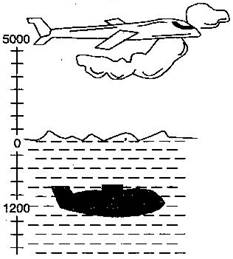Answer: Height of a plane above the sea level = 5000 m

Floating a submarine below the sea level = 1200 m

$\therefore$ The vertical distance between the plane and the submarine = 5000 + 1200 = 6200 m

Thus, the vertical distance between the plane and the submarine is 6200 m.

Question 5. Mohan deposits Rs. 2,000 in his bank account and withdraws Rs. 1,642 from it, the next day. If withdrawal of amount from the account is represented by a negative integer, then how will you represent the amount deposited? Find the balance in Mohan’s accounts after the withdrawal?

Answer: Deposit amount = Rs. 2,000 and Withdrawal amount = Rs. 1,642

$\therefore$ Balance = 2,000 – 1,642 = Rs. 358

Thus, the balance in Mohan’s account after withdrawal is Rs. 358.

Question 6. Rita goes 20 km towards east from a point A to the point B. From B, she moves 30 km towards west along the same road. If the distance towards east is represented by a positive integer then, how will you represent the distance travelled towards west? By which integer will you represent her final position from A?

Answer : According to the number line, Rita moves towards east is represented by a positive integer. But she moves in opposite direction means Rita moves west, is represented by negative integer.

Distance from A to B = 20 km

Distance from B to C = 30 km

Distance from A to C = 20 – 30 = –10 km

Thus, Rital is at final position from A to C is –10 km.

Question 7. In a magic square each row, column and diagonal have the same sum. Check which of the following is a magic square.

(i)

 5 -1 4 -5 -2 7 0 3 -3

(ii)

 1 -10 0 -4 -3 -2 -6 4 -7

Answer: (i) Taking rows 5 + (–1) + (–4) = 5 – 5 = 0

(–5) + (–2) + 7 = –7 + 7 = 0

0 + 3 + (–3) = 3 – 3 = 0

Taking columns 5 + (–5) + 0 = 5 – 5 = 0

(–1) + (–2) + 3 = –3 + 3 = 0

(–4) + 7 + (–3) = 7 – 7 = 0

Taking diagonals 5 + (–2) + (–3) = 5 – 5 = 0

(–4) + (–2) + 0 = –6

This box is not a magic square because all the sums are not equal.

(ii) Taking rows 1 + (–10) + 0 = 1 – 10 = –9

(–4) + (–3) + (–2) = –7 – 2 = –9

(–6) + 4 + (–7) = –2 – 7 = –9

Taking columns 1 + (–4) + (–6) = 1 – 10 = –9

(–10) + (–3) + 4 = –13 + 4 = –9

0 + (–2) + (–7) = 0 – 9 = –9

Taking diagonals 1 + (–3) + (–7) = 1 – 10 = –9

0 + (–3) + (–6) = –9

This box is magic square because all the sums are equal.

Question 8.Verify $a-\left(-b\right)=a+b$ for the following values of $a$ and $b:$

(i) $a=21,b=18$

(ii) $a=118,b=125$

(iii) $a=75,b=84$

(iv) $a=28,b=11$

Answer: (i) Given: $a=21,b=18$

We have $a-\left(-b\right)=a+b$

Putting the values in L.H.S. = $a-\left(-b\right)=21-\left(-18\right)$ = 21 + 18 = 39

Putting the values in R.H.S. = $a+b$ = 21 + 19 = 39

Since, L.H.S. = R.H.S Hence, verified.

(ii) Given: $a=118,b=125$

We have $a-\left(-b\right)=a+b$

Putting the values in L.H.S. = $a-\left(-b\right)=118-\left(-125\right)$ = 118 + 125 = 243

Putting the values in R.H.S. = $a+b$ = 118 + 125 = 243

Since, L.H.S. = R.H.S Hence, verified.

(iii) Given: $a=75,b=84$

We have $a-\left(-b\right)=a+b$

Putting the values in L.H.S. = $a-\left(-b\right)=75-\left(-84\right)$ = 75 + 84 = 159

Putting the values in R.H.S. = $a+b$ = 75 + 84 = 159

Since, L.H.S. = R.H.S Hence, verified.

(iv) Given: $a=28,b=11$

We have $a-\left(-b\right)=a+b$

Putting the values in L.H.S. = $a-\left(-b\right)=28-\left(-11\right)$ = 28 + 11 = 39

Putting the values in R.H.S. = $a+b$ = 28 + 11 = 39

Since, L.H.S. = R.H.S Hence, verified.

Question 9. Use the sign of >, < or = in the box to make the statements true:

(a)

(b)

(c)

(d)

(e)

Answer: (a)  $⇒$

$⇒$  $⇒$

(b)  $⇒$

$⇒$  $⇒$

$⇒$

(c) $⇒$

$⇒$  $⇒$

(d) $⇒$

$⇒$  $⇒$

$⇒$

(e) $⇒$

$⇒$  $⇒$

Question 10. A water tank has steps inside it. A monkey is sitting on the topmost step (i.e., the first step). The water level is at the ninth step: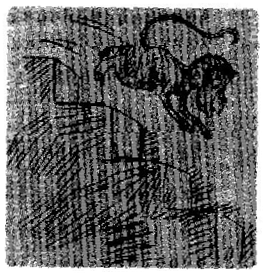(i) He jumps 3 steps down and then jumps back 2 steps up. In how many jumps will he reach the water level?

(ii) After drinking water, he wants to go back. For this, he jumps 4 steps up and then jumps back 2 steps down in every move. In how many jumps will he reach back the top step?

(iii) If the number of steps moved down is represented by negative integers and the number of steps move up by positive integers, represent his moves in part (i) and (ii) by completing the following:
(a) $-3+2-..........=-8$

(b) $4-2+........=8$

In (a) the sum $\left(-8\right)$ represent going down by eight steps. So, what will the sum 8 in (b) represent?

Answer: (i) He jumps 3 steps down and jumps back 2 steps up. Following number ray shows the

jumps of monkey: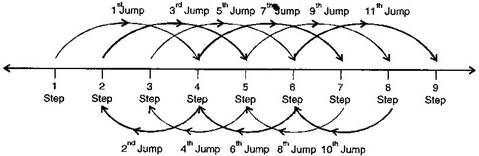First jump = 1 + 3 = 4 steps Second jump = 4 – 2 = 2 steps

Third jump = 2 + 3 = 5 steps Fourth jump = 5 – 2 = 3 steps

Fifth jump = 3 + 3 = 6 steps Sixth jump = 6 – 2 = 4 steps

Seventh jump = 4 + 3 = 7 steps Eighth jump = 7 – 2 = 5 steps

Ninth jump = 5 + 3 = 8 steps Tenth jump = 8 – 2 = 6 steps

Eleventh jump = 6 + 3 = 9 steps

He will reach ninth steps in 11 jumps.

(ii) He jumps four steps and them jumps down 2 steps. Following number ray shows the

jumps of monkey: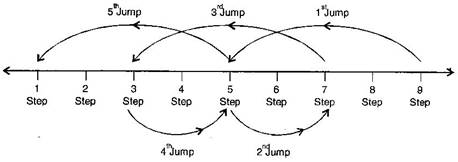Thus monkey reach back on the first step in fifth jump.

(iii) (a) $-3+2-3+2-3+2-3+2-3+2-3+2-3+2-3+2=-8$

(b) $4-2+4-2+4-2+4-2=8$

Thus, sum 8 in (b) represents going up by eight steps.